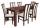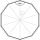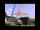# Planimetrics - math word problems

Study plane measurements, including angles, distances, and areas. In other words - measurement and calculation of shapes in the plane. Perimeter and area of plane shapes.

1. Perimeter to areaCalculate the area of a circle with the perimeter 15 meters.What is the diameter of a tree around which is 3m 9dm 7cm long rope wrapped? How big is its cross-sectional area?
3. RT perimeterThe leg of the rectangular triangle is 7 cm shorter than the second leg and 8 cm shorter than the hypotenuse. Calculate the triangle circumference.
4. TableThe circumference of the rectangle table is 420 cm. Length to width ratio is 5:2 . Calculate table dimensions and dimensions reduced in the ratio 3:5
5. Square glassWhat is the area of the square window glass with the side 4 dm? Do you know how many cm2 is it?
6. Trapezoid - central medianThe central median divides the trapezoid into two smaller trapezoids. Determines the ratio of their contents.
7. Cross sectionThe cross-section ABCD of a swimming pool is a trapezium. Its width AB=14 meters, depth at the shallow end is 1.5 meters, and at the deep end is 8 meters. Find the area of the cross-section.
8. Rectangle 35Find the area of a rectangle when the diagonal is equal to 30 cms and the width is double the length.
9. The rectangleDetermine the area of the rectangle where the length and width are in the ratio 5:2 and its length is 7.5 cm longer than its width. Determine also its length and its width.On a straight stretch of road is marked 12 percent drop. What angle makes the direction of the road with the horizontal plane?
11. Medians of isosceles triangleThe isosceles triangle has a base ABC |AB| = 16 cm and 10 cm long arm. What are the length of medians?
12. Regular n-gonIn a regular n-angle polygon the internal angle is 144 degrees. Find the number n indicating the number of sides of this polygon.
13. Two aircraftFrom the airport will start simultaneously two planes, which fly tracks are perpendicular to each other. The first flying speed of 680 km/h and the second 840 km/h. Calculate how far the aircraft will fly for half an hour.
14. StairwayStairway has 20 steps. Each step has a length of 22 cm and a height of 15 cm. Calculate the length of the handrail of staircases if on the top and bottom exceeds 10 cm.
15. A mastA mast 32 meters high was broken by the wind so that its top touches the ground 16 meters from the pole. The still standing part of the mast, the broken part and the ground form a rectangular triangle. At what height was the mast broken?
16. Rhombus MATHConstruct a rhombus M A T H with diagonal MT=4cm, angle MAT=120°
17. Plane IIA plane flew 50 km on a bearing 63degrees20 and the flew on a bearing 153degrees20 for 140km. Find the distance between the starting point and the ending point
18. RectangleCalculate area of the rectangle if its length is 12 cm longer than its width and length is equal to the square of its width.
19. Rectangular triangle PQRIn the rectangular triangle PQR, the PQ leg is divided by the X point into two segments of which longer is 25cm long. The second leg PR has a length 16 cm. The length of the RX is 20 cm. Calculate the length p of side RQ. The result is round to 2 decimalThe double ladder shoulders should be 3 meters long. What height will the upper top of the ladder reach if the lower ends are 1.8 meters apart?